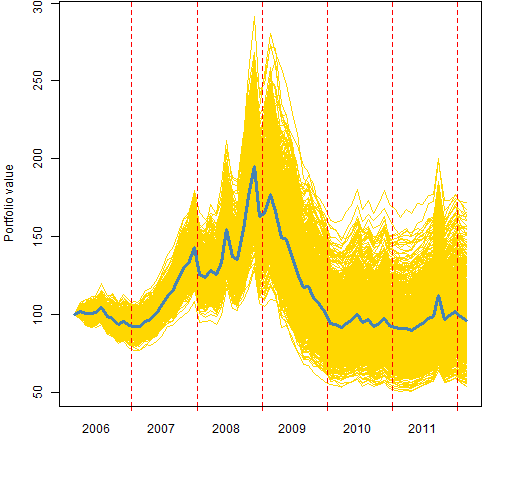# Again with variability of long-short decile tests

A simpler approach to producing the variability.

## Previously

The post “Variability in long-short decile strategy tests” proposed a way of assessing the variability of strategy tests in which a long-short portfolio is created by equally weighting the top and bottom deciles.

## Improved idea

Joe Mezrich suggests maintaining equal weights but bootstrapping the assets within the deciles.  For me that passes the jealousy test — why didn’t I think of that?

It eliminates the arbitrariness of the selection of the range of weights to use in the previous method.

Figure 1 shows the variability of 1000 bootstrap paths for the MACD signal that the previous post used.

Figure 1: Efficacy of MACD via long-short deciles with bootstrapped equal weights.The bootstrap variability is bigger than the biggest of the variabilities investigated previously.

## Appendix R

The function to do the bootstrapping is a very mildly revised version of the function that does the random weighting.

```pp.decileTestBoot <-
function(signal, prices, trials=1000, groups=10)
{
# R function to test a signal
# via long-short deciles
# and bootstrapping the deciles

# put in the public domain 2012 by Burns Statistics

# testing status:
# seems to work

stopifnot(all(dim(signal) == dim(prices)),
length(groups) == 1,
identical(sort(colnames(signal)),
sort(colnames(prices))))

ntimes <- nrow(prices)
eqwtval <- rep(NA, ntimes)
names(eqwtval) <- rownames(prices)
randwtval <- array(NA, c(length(eqwtval), trials),
list(names(eqwtval), NULL))
uret <- tail(prices, -1) / head(prices, -1) - 1
randwtval[1,] <- eqwtval <- 100

nside <- round(ncol(prices) / groups)

if(trials) {
tseq <- 1:trials
t.eret <- numeric(trials)
}

for(i in 1:(ntimes-1)) {
tb <- pp.topBottom(signal[i, ], n=nside)
botret <- uret[i, tb\$bottom]
topret <- uret[i, tb\$top]
this.eret <- mean(topret) - mean(botret)
eqwtval[i+1] <- eqwtval[i] * (1 + this.eret)
if(trials) {
for(j in tseq) {
t.eret[j] <- mean(sample(topret, nside,
replace=TRUE)) - mean(sample(botret,
nside, replace=TRUE))
}
randwtval[i+1, ] <- randwtval[i, ] *
(1 + t.eret)
}
}
ans <- list(equal.weight=eqwtval,
random.weight=randwtval, nside=nside,
call=match.call())
class(ans) <- "SignalTest"
ans
}```
This entry was posted in Quant finance, R language and tagged , . Bookmark the permalink.